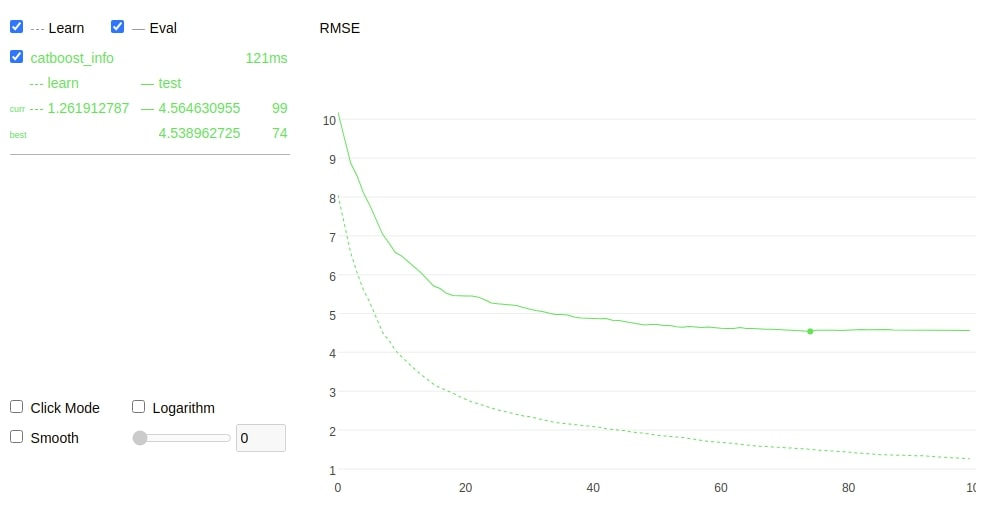# CatBoost: 機器學習 boosting 神器介紹

2021/10/01

## 前言## CatBoost 優點

CatBoost 名稱源於 Category 和 boost 兩個單詞，承襲 boosting 的優點之外該演算法在類別型的特徵上做了一些更公平的特徵工程。訓練過程中允許沒有編碼的類別特徵，透過分類和數字特徵組合的各種統計量為類別型的特徵做編碼。不過在訓練前必須確保該特徵中無缺失值。其訓練資料若有缺失值 CatBoost 預設會將數值型的資料補上最小值，詳細內容可以參考。另外對於 GPU 的使用者，它也能處理字串(類別)型態的特徵。

• 自動處理類別型的特徵
• 自動處理缺失值
• 可以處理各種數據類型，如音頻、文字、圖像
• 減少人工調參的需要，並降低了過擬合的機會

## CatBoost 安裝

CatBoost 演算法可以解決分類(CatBoostClassifier)和回歸(CatBoostRegressor)的問題。安裝的方式也非常簡單，使用 `pip` 就能輕鬆安裝。

``````pip install catboost
``````

## CatBoost Parameters

CatBoost 基本上可以自由的讓演算法去選擇最佳的模型，不過 API 還是提供一些基本的超參數讓使用者手動調整。

Parameters:

• iterations: 總共迭代的次數，即決策樹的個數。預設值為1000。
• use_best_model: 設定 True 時必須給定驗證集，將會留下驗證集分中數最高的模型。
• early_stopping_rounds: 連續訓練N代，若結果未改善則提早停止訓練。
• od_type: IncToDec/Iter，預設 Iter 防止 Overfitting 評估方式，若設定前者需要設定閥值。
• eval_metric: 模型評估方式。
• loss_function: 計算loss方法。
• verbose: True(1)/Flase(0)，預設1顯示訓練過程。
• random_state: 亂數種子，確保每次訓練結果都一樣。
• learning_rate: 預設 automatically。
• depth: 樹的深度，預設6。
• cat_features: 輸入類別特徵的索引，它會自動幫你處理。

Attributes:

• feature_importances_: 查詢模型特徵的重要程度。

Methods:

• fit: 放入X、y進行模型擬合。
• predict: 預測並回傳預測類別。
• score: 預測成功的比例。

## 模型訓練

``````from catboost import CatBoostRegressor

# 建立模型
model = CatBoostRegressor(random_state=42,
loss_function='RMSE',
eval_metric='RMSE',
use_best_model=True)
# 使用訓練資料訓練模型
model.fit(X_train,y_train, eval_set=(X_test, y_test), verbose=0, plot=True)
``````## 特徵篩選

``````from catboost import CatBoostRegressor, Pool, EShapCalcType, EFeaturesSelectionAlgorithm

# feature_names = ['F{}'.format(i) for i in range(X_train.shape)]
train_pool = Pool(X_train, y_train, feature_names=boston_dataset.feature_names.tolist())
test_pool = Pool(X_test, y_test, feature_names=boston_dataset.feature_names.tolist())

model = CatBoostRegressor(random_state=42,
loss_function='RMSE',
eval_metric='RMSE',
use_best_model=True)
summary = model.select_features(
train_pool,
eval_set=test_pool,
features_for_select='0-12',
num_features_to_select=3,
steps=2,
algorithm=EFeaturesSelectionAlgorithm.RecursiveByShapValues,
shap_calc_type=EShapCalcType.Regular,
train_final_model=True,
logging_level='Silent',
plot=False
)
summary
``````

``````{'selected_features': [5, 10, 12],
'eliminated_features_names': ['DIS',
'B',
'ZN',
'CHAS',
'INDUS',
'CRIM',
'AGE',
'TAX',
'NOX'],
'eliminated_features': [7, 11, 1, 3, 8, 2, 0, 6, 9, 4],
'selected_features_names': ['RM', 'PTRATIO', 'LSTAT']}
``````

``````from catboost import CatBoostRegressor
grid = {'iterations': [100, 150, 200],
'learning_rate': [0.03, 0.1],
'depth': [2, 4, 6, 8],
'l2_leaf_reg': [0.2, 0.5, 1, 3]}

model = CatBoostRegressor(random_state=42,
loss_function='RMSE',
eval_metric='RMSE')
model.grid_search(grid, X_train,y_train)
``````

## 自動處理類別型的特徵

CatBoost 無需對數據特徵進行任何的預處理就可以將類別轉換爲數字。下面程式為一個分類問題的範例，其中輸入特徵的第一個為季節。在機器學習上的認知我們必須將所以字串型資料必須透過標籤編碼方式轉換成數值，然而在 CatBoost 完全不需要。僅需在訓練模型時給予參數 `cat_features = ` 即代表資料的第一個特徵需要進行類別轉換。另外輸出葉不一定要編碼後的結果，你也可以丟入文字進行訓練只要加上 `loss_function='MultiClass'` 即可。

``````from catboost import Pool, CatBoostClassifier

train_data = [["summer", 1924, 44],
["summer", 1932, 37],
["winter", 1980, 37],
["summer", 2012, 204]]

eval_data = [["winter", 1996, 197],
["winter", 1968, 37],
["summer", 2002, 77],
["summer", 1948, 59]]

train_label = ["France", "USA", "USA", "UK"]
eval_label = ["USA", "France", "USA", "UK"]

# Initialize CatBoostClassifier
model = CatBoostClassifier(iterations=10,
learning_rate=1,
depth=2,
cat_features = ,
loss_function='MultiClass')
# Fit model
model.fit(train_data, train_label)
# Get predicted classes
preds_class = model.predict(eval_data)
# Get predicted probabilities for each class
preds_proba = model.predict_proba(eval_data)
# Get predicted RawFormulaVal
preds_raw = model.predict(eval_data,
prediction_type='RawFormulaVal')
``````

## 善用 Verbose## 模型的解釋

CatBoost 提供了 `plot` 可以方便在訓練時查看並作即時分析訓練趨勢。除此之外 CatBoost 也支援 SHAP 增加了模型可解釋。詳細的使用方式可以參考官方教學## 小結

CatBoost 的優點和使用方法都介紹完了，是不是覺得十分簡單易用且功能強大。尤其是遇到資料需要大量處理和特徵數值化的任務時最適合使用 CatBoost 了。真的是所謂的懶人套件，名符其實的 Ying Train Yi Fa(硬Train一發)!## Referencehttps://www.youtube.com/channel/UCSNPCGvMYEV-yIXAVt3FA5A